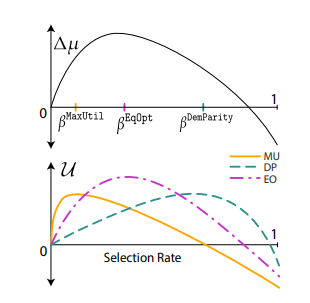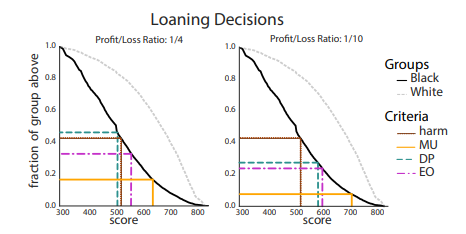## Master X Blog

ECNU & HUST Student | Amateur Author

# Delayed Impact of Fair Machine Learning

## 背景设置

1）不受约束：银行只考虑尽可能最大化利润时信用评分的阈值，用户达到即可放贷。

2）人口均等（选择率均等）：银行以相同利率贷款给两组，利率的设置使银行尽可能最大化利润。

3）最初机会相等（真阳率）：两组之间实际利率相等，对每个用户的标准均相同的情况下，尽可能最大化利润

## 问题设置

$u(\tau)=\sum_{j∈{A,B}}g_j \sum_{x∈\chi}\tau_j(x)\pi_j(x)u(x)$

$\Delta\mu_j(\tau):=\sum_{x∈\chi}\pi_j(x)\tau_j(x)\Delta(x)$

$\beta_j:=\sum_{x∈\chi}\pi_j(x)\tau_j(x)$1)不受约束（MaxUtil）：即只考虑阈值取值和效益

2)人口均等（选择EqOpt）：定义真阳率：

$TPR_j(\tau):=\frac{\sum_{x∈\chi}\pi_j(x)\rho(x)\tau(x)}{\sum_{x∈\chi}\pi_j(x)\rho(x)}$

$C={(\tau_A,\tau_B):TPR_A(\tau_A)=TPR_B(tau_B)}$

## 结果分析1）（公平标准会造成相对提升）假设$\beta_A^MaxUtil$ < $\hat{\beta}$$\beta_B^MaxUtil$>$\beta_A^MaxUtil$ ，那么存在群体比例g0<g1<1，使得对所有gA ∈ [g0, g1]，$\beta_A^MaxUtil$ < $\beta_A^DemParity$ < $\hat{\beta}$。即$\beta_A^DemParity$ 会有相对提升。

2）（公平标准会造成相对提升）假设存在 $\beta_A^MaxUtil$ < $\beta$ <$\beta^‘$ <$\hat{\beta}$  使得 $\beta_B^MaxUtil$ > $G^{(A→B)}(\beta)$$G^{(A→B)}(\beta^’)$ ，那么存在群体比例g2<g3<1，使得对所有gA ∈ [g2, g3]， $\beta_A^MaxUtil$ < $\beta_A^EqOpt$< $\hat{\beta}$$\beta_A^EqOpt$即 会有相对提升。

3）（人口均等过度会造成损失）对于选择率 $\beta$，假设 $\beta_B^MaxUtil$ > $\beta$$\beta_A^MaxUtil$。那么，存在比例g0使得对任意gA ∈ [0, g0]， $\beta_A^DemPatity$ $\beta$ 。特别地，当 $\beta$= $\beta_0$ 时，DemPatity会造成损害；当 $\beta$= $\hat{\beta}$  时，DemPatity会造成相对损失。

4）（机会相等过度会造成损失）假设$\beta_B^MaxUtil$>$G^{(A→B)}(\beta)$$\beta>\beta_A^MaxUtil$。那么，存在比例g0使对任意gA ∈ [0, g0]，$\beta>\beta_A^EqOpt$。特别地，当 $\beta>\beta_0$时，EqOpt会造成损害；当$\beta=\hat{\beta}$  时，EqOpt会造成相对损失。

5）（DemPatity永远不会借贷少于MaxUtil，但EqOpt会）假设$\beta_A^MaxUtil$ < $\beta_B^MaxUtil$$TPR_A(\tau^MaxUtil)$ >$TPR_B(\tau^MaxUtil)$ ，那么 $\beta_A^EqOpt$<$\beta_A^MaxUtil$<$\beta_A^DemPatity$。也就是EqOpt会造成相对损失。## 展望与个人分析

1.考虑超出群体平均变化影响的其他特征(如方差、个体水平结果)。

2.研究结果优化对建模和测量误差的鲁棒性